Monday 18th January 2021

CBSE Guess > Papers > Question Papers > Class XII > 2003 > Mathematics > Outside Delhi Set -III

MATHEMATICS—2003 (Set III—Outside Delhi)

Note: Except for the following questions, all the remaining questions have been asked in Set I & Set II .

SECTION - A

Q. 1. Evaluate: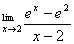Q. 7. Evaluate: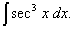Q. 11. Evaluate: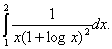Q. 13. In bag A there are 5 white and 8 red balls, in bag B, 7 white and 6 rd balls and in bag C, 6 white and 5 red balls. One ball is taken out at random from each bag. Find the probability that all the three balls are the same colour.

Q. 17. A square tank of capacity 250 cubic meters has to be dug out. The cost of the land is Rs. 50 per square meter. The cost of digging increases with the depth and for the whole tank it is Rs.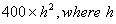meters is the depth of the tank. What should be the dimensions of the tank so that the cost be minimum?

Q. 20. Given that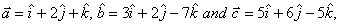verify that

Q. 21. The demand function of an output is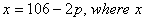is the number of units and P the price per unit, and the average cost per unit is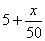, If the total revenue be PX, determine the number of units for maximum profit.

 Mathematics 2003 Question Papers Class XII Delhi Outside Delhi Compartment Delhi Compartment Outside DelhiSet ISet ISet ISet ISet IISet IISet IISet IISet IIISet III

CBSE 2003 Question Papers Class XII